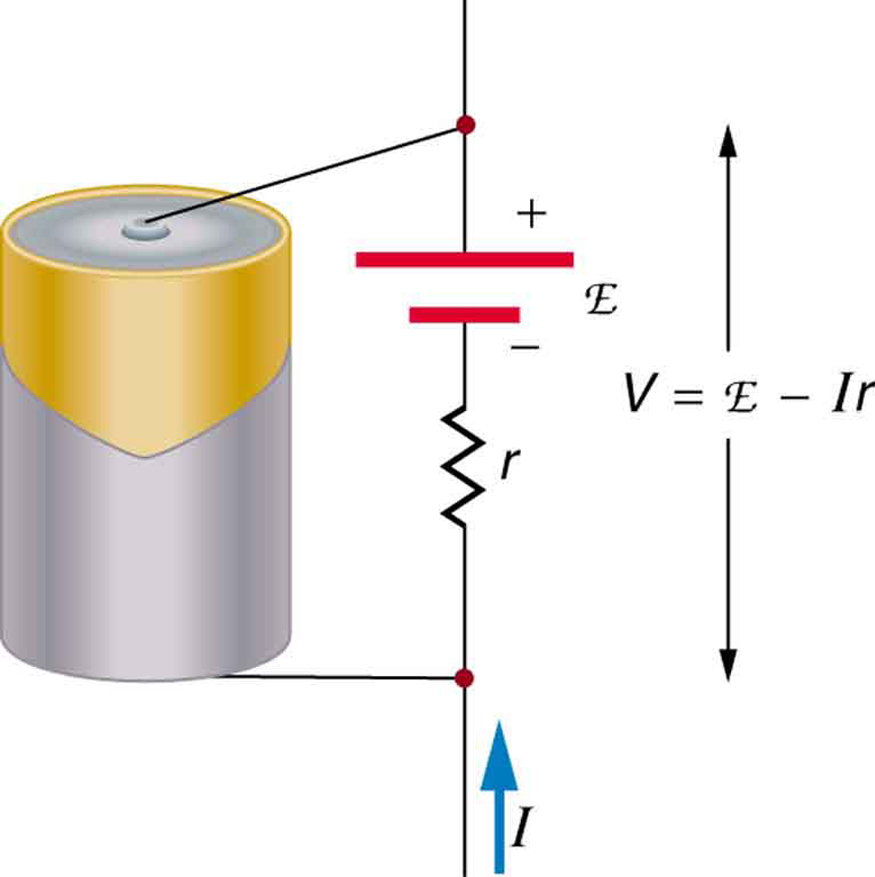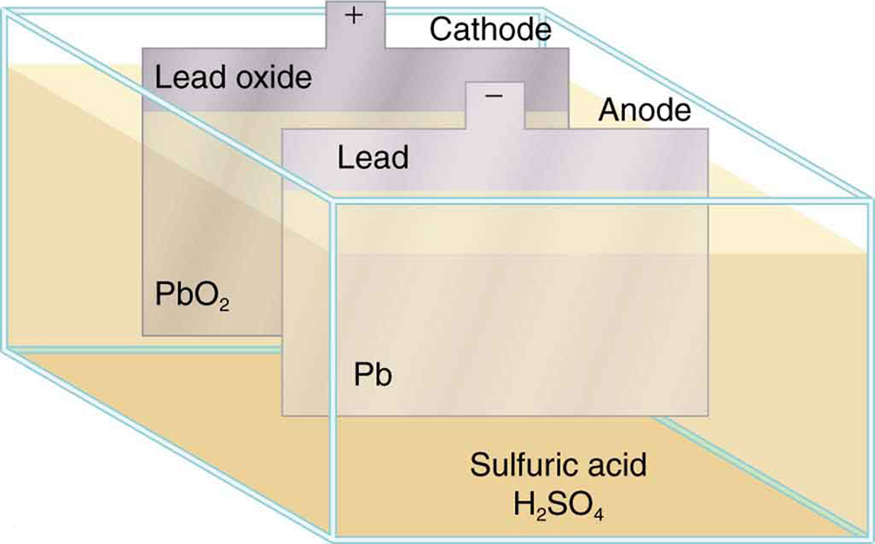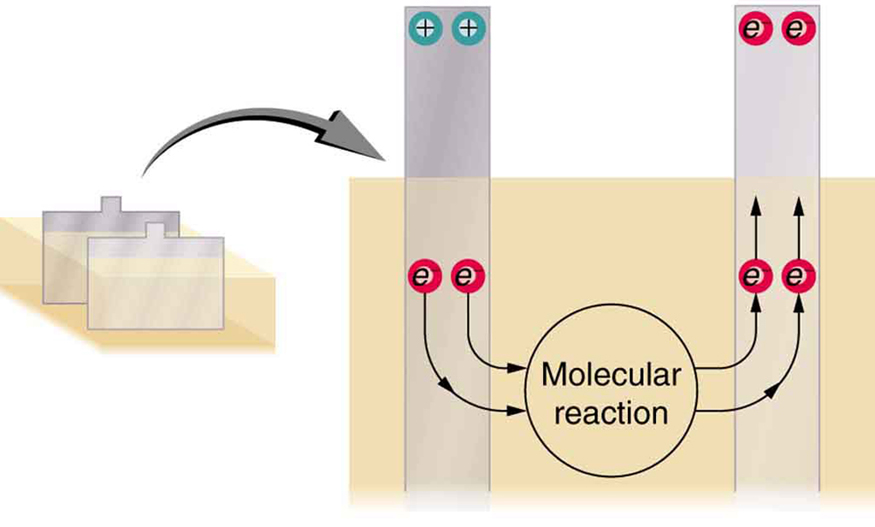# 21.2 Electromotive force: terminal voltage  (Page 2/12)

 Page 2 / 12

[link] is a schematic representation of the two fundamental parts of any voltage source. The emf (represented by a script E in the figure) and internal resistance $r$ are in series. The smaller the internal resistance for a given emf, the more current and the more power the source can supply.Any voltage source (in this case, a carbon-zinc dry cell) has an emf related to its source of potential difference, and an internal resistance r size 12{r} {} related to its construction. (Note that the script E stands for emf.). Also shown are the output terminals across which the terminal voltage V size 12{V} {} is measured. Since V = emf − Ir size 12{V="emf" - ital "Ir"} {} , terminal voltage equals emf only if there is no current flowing.

The internal resistance $r$ can behave in complex ways. As noted, $r$ increases as a battery is depleted. But internal resistance may also depend on the magnitude and direction of the current through a voltage source, its temperature, and even its history. The internal resistance of rechargeable nickel-cadmium cells, for example, depends on how many times and how deeply they have been depleted.

## Things great and small: the submicroscopic origin of battery potential

Various types of batteries are available, with emfs determined by the combination of chemicals involved. We can view this as a molecular reaction (what much of chemistry is about) that separates charge.

The lead-acid battery used in cars and other vehicles is one of the most common types. A single cell (one of six) of this battery is seen in [link] . The cathode (positive) terminal of the cell is connected to a lead oxide plate, while the anode (negative) terminal is connected to a lead plate. Both plates are immersed in sulfuric acid, the electrolyte for the system.Artist’s conception of a lead-acid cell. Chemical reactions in a lead-acid cell separate charge, sending negative charge to the anode, which is connected to the lead plates. The lead oxide plates are connected to the positive or cathode terminal of the cell. Sulfuric acid conducts the charge as well as participating in the chemical reaction.

The details of the chemical reaction are left to the reader to pursue in a chemistry text, but their results at the molecular level help explain the potential created by the battery. [link] shows the result of a single chemical reaction. Two electrons are placed on the anode, making it negative, provided that the cathode supplied two electrons. This leaves the cathode positively charged, because it has lost two electrons. In short, a separation of charge has been driven by a chemical reaction.

Note that the reaction will not take place unless there is a complete circuit to allow two electrons to be supplied to the cathode. Under many circumstances, these electrons come from the anode, flow through a resistance, and return to the cathode. Note also that since the chemical reactions involve substances with resistance, it is not possible to create the emf without an internal resistance.Artist’s conception of two electrons being forced onto the anode of a cell and two electrons being removed from the cathode of the cell. The chemical reaction in a lead-acid battery places two electrons on the anode and removes two from the cathode. It requires a closed circuit to proceed, since the two electrons must be supplied to the cathode.

What is the difference between a principle and a law
the law is universally proved. The principal depends on certain conditions.
Dr
it states that mass of an element deposited during electrolysis is directly proportional to the quantity of electricity discharge
Olamide
what does the speedometer of a car measure ?
Car speedometer measures the rate of change of distance per unit time.
Moses
describe how a Michelson interferometer can be used to measure the index of refraction of a gas (including air)
using the law of reflection explain how powder takes the shine off a person's nose. what is the name of the optical effect?
WILLIAM
is higher resolution of microscope using red or blue light?.explain
WILLIAM
what is dimensional consistent
Mohammed
In engineering and science, dimensional analysis is the analysis of the relationships between different physical quantities by identifying their base quantities and units of measure and tracking these dimensions as calculations or comparisons are performed
syed
can sound wave in air be polarized?
Unlike transverse waves such as electromagnetic waves, longitudinal waves such as sound waves cannot be polarized. ... Since sound waves vibrate along their direction of propagation, they cannot be polarized
Astronomy
A proton moves at 7.50×107m/s perpendicular to a magnetic field. The field causes the proton to travel in a circular path of radius 0.800 m. What is the field strength?
derived dimenionsal formula
what is the difference between mass and weight
assume that a boy was born when his father was eighteen years.if the boy is thirteen years old now, how is his father in
Isru
31yrs
Olamide
what is airflow
derivative of first differential equation
why static friction is greater than Kinetic friction
draw magnetic field pattern for two wire carrying current in the same direction
An American traveler in New Zealand carries a transformer to convert New Zealand’s standard 240 V to 120 V so that she can use some small appliances on her trip.
What is the ratio of turns in the primary and secondary coils of her transformer?
nkombo
what is energy
Yusuf
How electric lines and equipotential surface are mutually perpendicular?
The potential difference between any two points on the surface is zero that implies È.Ŕ=0, Where R is the distance between two different points &E= Electric field intensity. From which we have cos þ =0, where þ is the angle between the directions of field and distance line, as E andR are zero. Thus
sorry..E and R are non zero...

#### Get Jobilize Job Search Mobile App in your pocket Now!ByByBy OpenStaxBy Abby SharpBy OpenStaxBy OpenStaxBy Naveen TomarBy OpenStaxBy Mariah HauptmanBy OpenStaxBy Brooke DelaneyBy Subramanian Divya